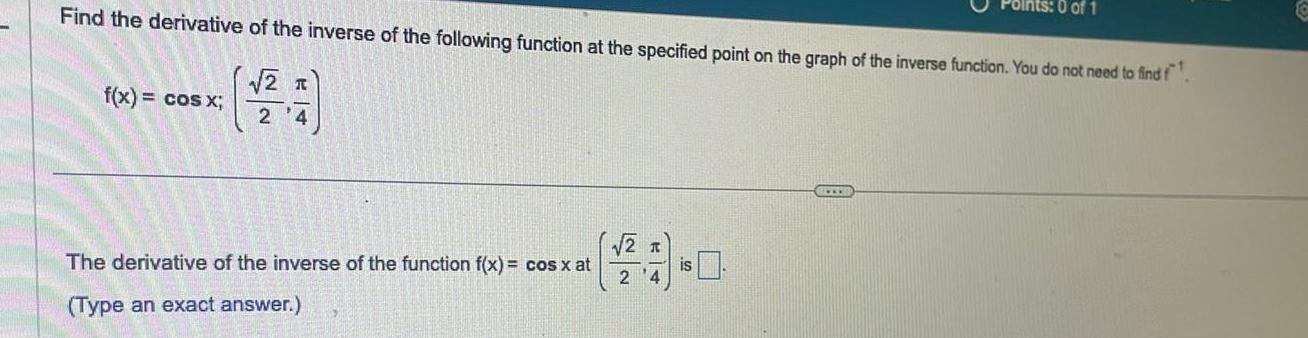Question:

# Find the derivative of the inverse of the following function

Last updated: 11/21/2023Find the derivative of the inverse of the following function at the specified point on the graph of the inverse function You do not need to find f 2 T 2 4 f x cos x The derivative of the inverse of the function f x cos x at Type an exact answer Points 0 of 1 2 T 24